# MCQ in DC Circuits Part 11 | REE Board Exam

(Last Updated On: November 13, 2020)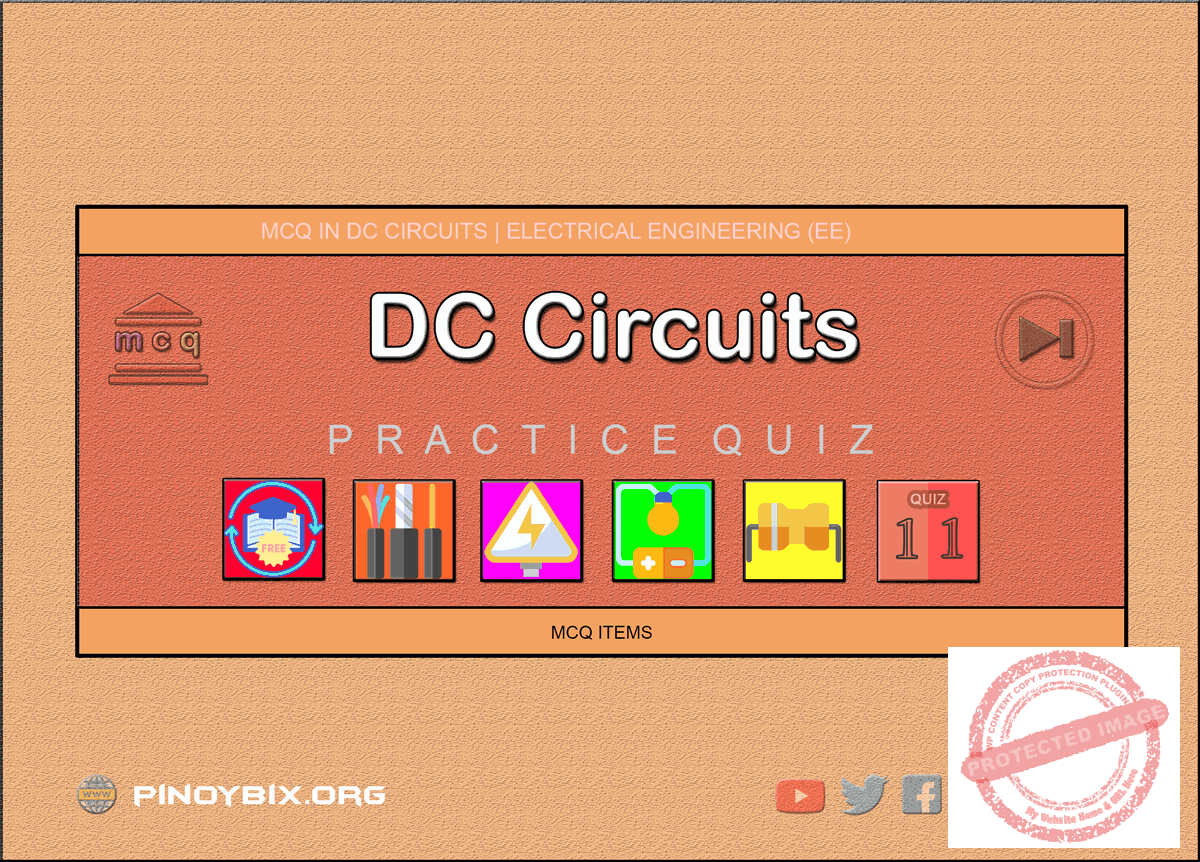This is the Multiple Choice Questions Part 11 of the Series in DC Circuits as one of the Electrical Engineering topic. In Preparation for the REE Board Exam make sure to expose yourself and familiarize in each and every questions compiled here taken from various sources including but not limited to past Board Exam Questions in Electrical Engineering field, Electrical Engineering Books, Journals and other Electrical Engineering References.

#### Continue Practice Exam Test Questions Part 11 of the Series

NETWORK THEOREMS

Choose the letter of the best answer in each questions.

501.  Determine  the  value  of  node  voltage  V2.  All resistances are in ohms.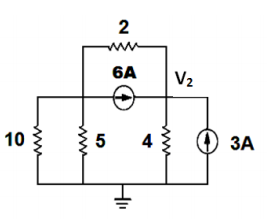A.  14 V

B.  12 V

C.  0 V

D.  1 V

Solution:

502.  What  should  be  the  value  of  R so  the  resistor  will receive the maximum power? All resistances are in ohms.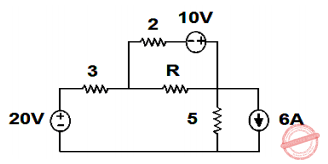A.  10.0 ohms

B.  3.875 ohms

C.  0.968 ohms

D.  1.60 ohms

Solution:

503.  Determine the value VO  in the ideal op-amp circuit below.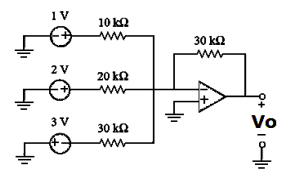A.  -8 V

B.  -6 V

C.  -4 V

D.  -3 V

Solution:

504.  Determine the value VO in the op-amp circuit below.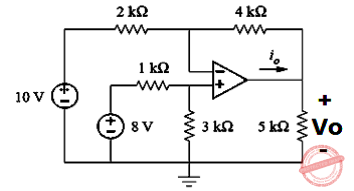A.  -4 V

B.  -8 V

C.  -2 V

D.  -3 V

Solution:

505.  If the voltage source (dependent or independent) is connected  between  two  non-reference  nodes,  the two non-reference nodes form a ______.

A.  Common Node

B.  Supernode

C.  Complex Node

D.  Reference node

Solution:

506.  The theorem that states that “the voltage across or current through an element in a linear circuit is the algebraic  sum  of  the  voltages  across  or  current through  that  element  due  to  each  independent source acting alone”.

A.  Superposition Theorem

B.  Thevenin’s Theorem

C.  Norton’s Theorem

D.  Reciprocity Theorem

Solution:

507.  Kirchhoff’s Current Law states that

A.  the  algebraic  sum  of  the  currents  flowing  into any point in a circuit must equal zero

B.  the algebraic sum of the currents  entering and leaving any point in a circuit must equal zero

C.  the algebraic sum of the currents flowing away from any point in a circuit must equal zero

D.  the  algebraic  sum  of  the  currents  around  any closed path must equal zero

Solution:

508.  When applying Kirchhoff’s Current Law,

A.  consider all the currents flowing into a branch point  positive  and  all  currents  directed  away from that point negative

B.  consider  all the  currents  flowing  into  a  branch point  negative  and  all  currents  directed  away from that point positive

C.  remember  that  the  total  of  all  the  currents entering a branch point must always be greater than the sum of the currents leaving that point

D.  the algebraic sum of the currents entering and leaving  a  branch  point  does  not  necessarily have to be zero

Solution:

509.  When  applying  Kirchhoff’s  Voltage  Law,  a  closed path is commonly referred to as a

A.  node

B.  principal node

C.  loop

D.  branch point

Solution:

510.  Kirchhoff’s Voltage Law states that

A.  the  algebraic  sum  of  the  voltage  sources  and IR voltage drops in any closed path must total zero

B.  the  algebraic  sum  of  the  voltage  sources  and IR  voltage  drops  around  any  closed  path  can never equal zero

C.  the  algebraic  sum  of  all  the  currents  flowing around any closed path must equal zero

D.  none of these

Solution:

511.  When applying Kirchhoff’s Voltage Law

A.  consider any voltage whose positive terminal is reached  first  as  negative  and  any  voltage whose  negative  terminal  is  reached  first  as positive

B.  always consider all voltage sources as positive and all resistor voltage drops as negative

C.  consider  any  voltage  whose  negative  terminal is  reached  first  as  negative  and  any  voltage whose  positive  terminal  is  reached  first  as positive

D.  always  consider  all  resistor  voltage  drops  as positive and all voltage sources as negative

Solution:

512.  The algebraic sum of +40 V and -30 V is

A.  -10 V

B.  +10 V

C.  +70 V

D.  -70 V

Solution:

513.  A principal node is

A.  a closed path or loop where the algebraic sum of the voltages must equal zero

B.  the  simplest  possible  closed  path  around  a circuit

C.  a  junction  where  branch  current  can  combine or divide

D.  none of these

Solution:

514.  How  many  equations  are  necessary  to  solve  a circuit with two principal nodes?

A.  3

B.  2

C.  4

D.  1

Solution:

515.  The  difference  between  a  mesh  current  and  a branch current is

A.  a  mesh  current  is  an  assumed  current  and  a branch current is an actual current

B.  the direction of the current themselves

C.  a  mesh  current  does  not  divide  at  a  branch point

D.  both A and B above

Solution:

516.  Using the method of mesh currents, any resistance common to two meshes has

A.  two opposing mesh currents

B.  one common mesh current

C.  zero current

D.  none of these

Solution:

517.  The fact that the sum of the resistor voltage drops equals the applied voltage in a series circuit is the basis for

A.  Kirchhoff’s Current Law

B.  node voltage analysis

C.  Kirchhoff’s Voltage Law

D.  the method of mesh currents

Solution:

518.  The  fact  that  the  sum  of  the  individual  branch currents equals the total current in a parallel circuit is the basis for

A.  Kirchhoff’s Current Law

B.  node voltage analysis

C.  Kirchhoff’s Voltage Law

D.  the method of mesh currents

Solution:

519.  If  you  do  not  go  completely  around  the  loop  when applying Kirchhoff’s Voltage Law, then

A.  the  algebraic  sum  of  the  voltages  will  always be positive

B.  the  algebraic  sum  is  the  voltage  between  the start and finish points

C.  the  algebraic  sum  of  the  voltages  will  always be negative

D.  the  algebraic  sum  of  the  voltages  cannot  be determined

Solution:

520.  A resistor is an example of a(n)

A.  bilateral component

B.  active component

C.  passive component

D.  both A and C

Solution:

521.  To  apply  Superposition  theorem,  all  components must be

A.  the active type

B.  both linear and bilateral

C.  grounded

D.  both nonlinear and unidirectional

Solution:

522.  When converting from a Norton-equivalent circuit to a Thevenin equivalent circuit or vice versa

A.  RN and RTH have the same value

B.  RN will always be larger than RTH

C.  IN is shorted-circuit to find VTH

D.  VTH is short-circuited to find IN

Solution:

523.  When  solving  for  the  Thevenin  equivalent resistance, RTH,

A.  all voltage sources must be opened

B.  all voltage sources must be short-circuited

C.  all  voltage  sources  must  be  converted  to current sources

D.  none of these

Solution:

524.  Thevenin’s  Theorem  states  that  an  entire  network connected  to  a  pair  of  terminals  can  be  replaced with

A.  a single current source in parallel with a single resistance

B.  a single voltage source in parallel with a single resistance

C.  a  single voltage source  in  series  with  a single resistance

D.  a  single  current  source  in  series  with  a  single resistance

Solution:

525.  Norton’s  Theorem  states  that  an  entire  network connected  to  a  pair  of  terminals  can  be  replaced with

A.  a single current source in parallel with a single resistance

B.  a single voltage source in parallel with a single resistance

C.  a  single voltage source  in  series  with  a single resistance

D.  a  single  current  source  in  series  with  a  single resistance

Solution:

526.  With  respect  to  terminals  A  and  B  in  a  complex network, the Thevenin voltage, VTH, is

A.  the  voltage  across  terminals  A  and  B  when they are short-circuited

B.  the open-circuit voltage across terminals A and B

C.  the same as the voltage applied to the complex network

D.  none of these

Solution:

527.  With  respect  to  terminals  A  and  B  in  a  complex network, the Norton current, IN, is

A.  the  current flowing between terminals A and B when they are open

B.  the total current supplied by the applied voltage to the network

C.  zero  when  terminals  A  and  B  are  short-circuited

D.  the  current  flowing  terminals  A  and  B  when they are short-circuited

Solution:

528.  Which  theorem  provides  a  shortcut  for  finding  the common  voltage  across  any  number  of  parallel branches with different sources?

A.  The Superposition Theorem

B.  Thevenin’s Theorem

C.  Norton’s Theorem

D.  Millman’s Theorem

Solution:

529.  A d.c. circuit usually has ____ as the load

A.  Resistance

B.  Capacitance

C.  Inductance

D.  both inductance and capacitance

Solution:

530.  Electrical  appliances  are  connected  in  parallel because it ____.

A.  is a simple circuit

B.  draws less current

C.  results in reduce in power loss

D.  makes the operation of appliances independent of each other

Solution:

531.  The purpose of load in an electric circuit is to ____

A.  increase the circuit current

B.  utilize electrical energy

C.  decrease the circuit current

D.  none of these

Solution:

532.  A passive network has

A.    no emf source

B.    no current source

C.  neither emf nor current source

D.  none of these

Solution:

533.  The relationship between voltage and current is the same for two opposite directions of current in case of

A.    bilateral network

B.    active network

C.  unilateral network

D.  passive network

Solution:

534.  Which of the following statement is not correct?

A.    voltage source is an active element

B.    current source is a passive element

C.  resistance is a passive element

D.  conductance is a passive element

Solution:

535.  A resistance R is connected across two batteries, A and B connected in parallel. The open circuit emfs and internal resistances of the batteries are 12 V, 2 ohms  and  8  V,  1  ohm  respectively.  Determine  the ohmic  value  of  R  if  the  power  absorbed  by  R  is 7.656 watts.

A.  10 Ω

B.  12 Ω

C.  9 Ω

D.  8 Ω

Solution:

536.  A  network  has  7  nodes  and  5  independent  loops. The number of branches in the network is

A.  13

B.  12

C.  11

D.  10

Solution:

537.  The nodal method of circuit analysis is based on

A.  Kirchhoff’s Voltage Law & Ohm’s law

B.  Kirchhoff’s Current Law & Ohm‘s law

C.  Kirchhoff’s  Current  Law  &  Kirchhoff’s  Voltage Law

D.  Kirchhoff’s  Current  Law  &  Kirchhoff’s  Voltage Law & Ohm‘s law

Solution:

538.  For  a  network  of  seven  branches  and  four  nodes, the number of independent loops will be

A.  11

B.  8

C.  7

D.  4

Solution:

539.  A  network  has  b  branches  and  nodes.  For  this mesh analysis will be simpler then node analysis if n is greater then

A.  b

B.  b + 1

C.  (b/2) + 1

D.  b/2

Solution:

540.  The number of independent loops for a network with n nodes and b branches is

A.  n – 1

B.  b – n

C.  b – n + 1

D.  independent no. of nodes

Solution:

541.  The following constitutes a bilateral element

A.  Resistor

B.  FET

C.  Vacuum Tube

D.  metal rectifier

Solution:

542.  Kirchhoff’s Laws fail in the case of

A.  linear networks

B.  non-linear networks

C.  dual networks

D.  distributed parameter networks

Solution:

543.  Ohm’s  law,  Kirchhoff’s  Current  Law  &  Kirchhoff’s Voltage will fail at

A.  Low frequency

B.  high frequency

C.  high power

D.  none of these

Solution:

544.  Total no, of mesh equations required is equal to

B.  number of tree branches

C.  number of nodes

D.  none of these

Solution:

545.  The  minimum  number  of  equations  required  to analyze the circuit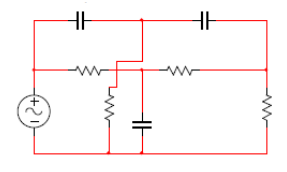A.  3

B.  4

C.  6

D.  7

Solution:

546.  Equivalent impedance seen across terminals a, b is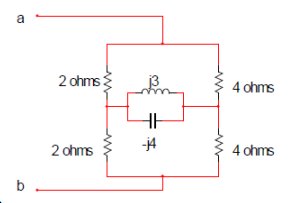A.  16/3 Ω

B.  8/3 Ω

C.  8/3 + j12

D.  none of these

Solution:

547.  What  is  the  Rab  in  the  circuit  when  all  resistors values are R?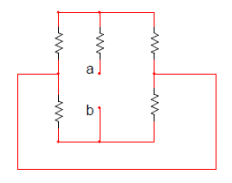A.  2R

B.  R

C.  R/2

D.  3R

Solution:

548.  Find Rab. All values are in ohms.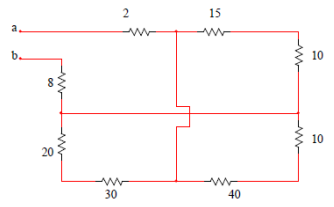A.  22.5

B.  40

C.  30

D.  none of these

Solution:

549.  Find  the  equivalent  resistance  of  the  circuit  in  the figure.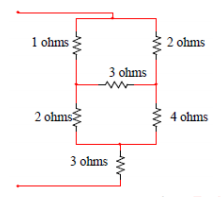A.  3 ohms

B.  4 ohms

C.  5 ohms

D.  6 ohms

Solution:

550.  Find  the  equivalent  resistance  of  the  circuit  in  this figure.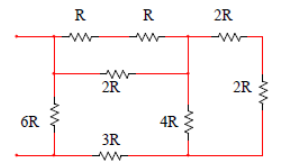A.  R

B.  2R

C.  3R

D.  4R

Solution:

#### Questions and Answers in DC Circuits

Following is the list of practice exam test questions in this brand new series:

MCQ in DC Circuits
PART 1: MCQ from Number 1 – 50                Answer key: included
PART 2: MCQ from Number 51 – 100            Answer key: included
PART 3: MCQ from Number 101 – 150           Answer key: included
PART 4: MCQ from Number 151 – 200           Answer key: included
PART 5: MCQ from Number 201 – 250           Answer key: included
PART 6: MCQ from Number 251 – 300           Answer key: included
PART 7: MCQ from Number 301 – 350           Answer key: included
PART 8: MCQ from Number 351 – 400            Answer key: included
PART 9: MCQ from Number 401 – 450            Answer key: included
PART 10: MCQ from Number 451 – 500          Answer key: included

P inoyBIX educates thousands of reviewers and students a day in preparation for their board examinations. Also provides professionals with materials for their lectures and practice exams. Help me go forward with the same spirit.

“Will you subscribe today via YOUTUBE?”

Subscribe

PinoyBIX Engineering. © 2014-2020 All Rights Reserved | How to Donate? |